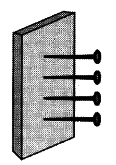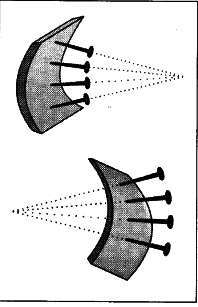# Explain an activity to find the normal to a curved surface

1. Take a small piece of thin foam or rubber.
2. Put some pins in a straight line on the foam.
3. All these pins are perpendicular to the foam.
4. If the foam was considered as a mirror, each pin would represent the normal at the point.5. Any ray incident at the point where the pin makes contact with the surface will reflect at the same angle the incident ray made with the pin - normal.
6. Now bend the foam piece inwards.
7. The pins still represent the normal at various points.
8. You will observe that all the pins tend to coverage at a point.
9. This will be appear like a concave mirror.
10. Now bend the foam piece outwards.
11. The pins seem to move away from each other that means they diverge.
12. The pins still represent the normal at various points.
13. This will be appear like a convex mirror.